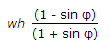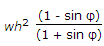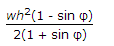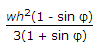# Civil Engineering - RCC Structures Design

### Exercise :: RCC Structures Design - Section 5

21.

The weight of reinforced concrete, is generally taken as

 A. 2200 kg/m3 B. 2300 kg/m3 C. 2400 kg/m3 D. 2500 kg/m3 E. 2800 kg/m3

Explanation:

No answer description available for this question. Let us discuss.

22.

Total pressure on the vertical face of a retaining wall of height h per unit run exerted by the retained earth weighing w per unit volume, is

 A.B.C.D.Explanation:

No answer description available for this question. Let us discuss.

23.

The zone in which transverse bending is likely to occur may be obtained by drawing a line from the faces of the column making an angle θ° with horizontal where θ° is

 A. 30° B. 45° C. 60° D. none of these.

Explanation:

No answer description available for this question. Let us discuss.

24.

If P kg/m2 is the upward pressure on the slab of a plain concrete footing whose projection on either side of the wall is a cm, the depth of foundation D is given by

 A. D = 0.00775 aP B. D = 0.0775 aP C. D = 0.07775 aP D. D = 0.775 Pa

Explanation:

No answer description available for this question. Let us discuss.

25.

According to load factor method, the permissible load W on a short column reinforced with longitudinal bars and lateral stirrups, is

 A. Stress in concrete x area of concrete B. Stress in steel x area of steel C. Stress in concrete x area of concrete + Stress in steel x area of steel D. None of these.